# How to Measure and Estimate a Roof Like a Pro? – DIY Guide with Diagrams!

What's a Typical Cost To Install a new Roof? Average Price: \$5,960 - \$12,740
See Costs Near You

Roofing is measured in squares. To figure out your roof’s area, a simple geometry can be used. The key is to be as accurate as possible since falling short, or an overage could mean thousands of dollars when planning your roofing budget.

## Need a Roofer?Get 4 Free Quotes From Local Pros:

Below, there is a simple triangle, and the math used to work out how to make a proper measurement. Once you work through the basic triangle, we will review a typical roof, and how to apply some simple math to calculate how much material you will need for a roof used in our hypothetical example.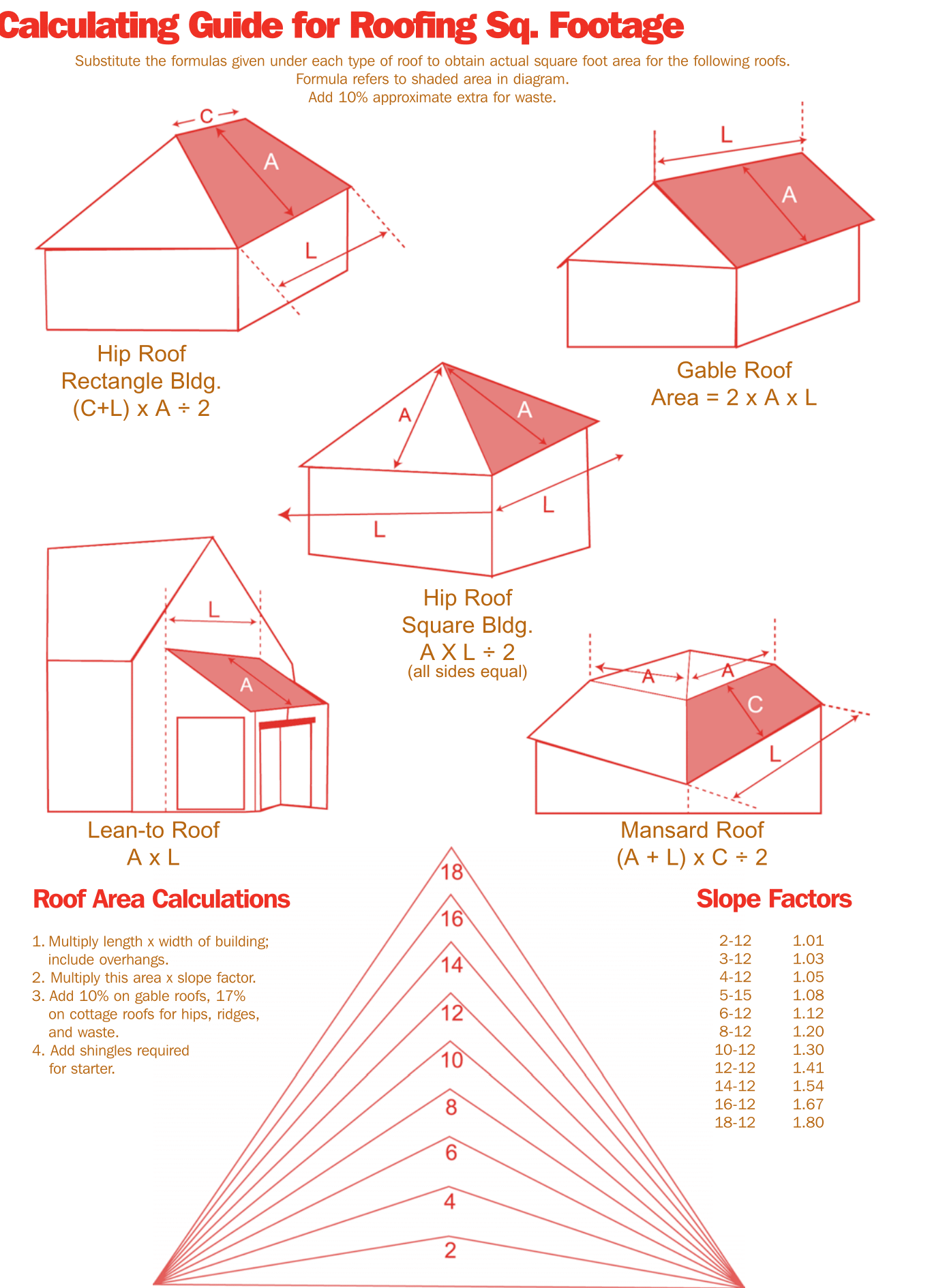1 roof square = 100 square feet; The length (l) times the height (h) of a triangle is twice its area (A2). So if you divide your answer of a product of length times height by two, you will get the area of a triangle. (l×h)/2 = Area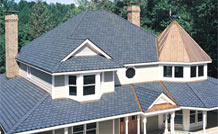New Shingle Roof \$7,500 Average price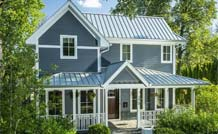New Metal Roof \$14,500 Average price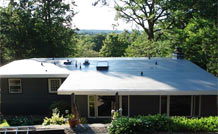New Flat Roof \$8,225 Average price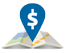See costs in your area Enter Your Zip Code

In Figure above: l=30 feet h=12 feet (30′ × 12′)/2 = 180 square feet

Now that the basic concept has been covered, let’s take a look at a more complex roofing system. In this overhead view you see both a hip-end section, and gable ends. With a more complex roof such is this one, it is highly recommended that you make a basic sketch. By doing so, it will be easier to mark your measurements, and calculate the necessary materials for the project. For this example we will break this roof up into sections labeled A through E.

Fig. A

Fig. B

In the figure B. above, a sketched portion is a basic triangle. As we did in the first example, measure the length of the eaves, and the vertical line from the eaves half way point to the peak. Multiply these numbers, and then divide the answer by two.
(30′ × 18′)/2 = 270 square feet

Fig. C

The easiest way to measure this section is to divide it up into three different sections: S1, S2, and S3. As you can see, S1 and S3 are the same size, but you still need to document all measurement points to verify your material calculation accuracy. Since S2 is now a rectangle, simple L x W is all that is required.

S1 = (18′ × 15′)/2 = 135 square feet
S2 = 50′ × 15′ = 750 square feet
S3 = (18′ × 15′)/2 = 135 square feet

Adding up our totals from these three sections = 1,020 square feet.

Fig. D

Again, we divide the roof up in sections. Measure eave to ridge and rake to valley for S4 and S5 sections. Section S6 and S7 are from the eave end to the valley end, as well as eave to ridge.

S6 = (18′ × 18′)/2 = 162 square feet
S7 = (18′ × 18′)/2 = 162 square feet
Or you could add the two 18′ widths, getting 36, and then multiply it by 18′ length, divide the answer by 2, and get the same answer of 324′ for both S6 and S7
For S1 and S2 we will add the total length on the rake side and multiply that by the eave to get our answer
18’+18’=36′ x 30’= 1080 square feet
Fig C total: 324′ + 1080′ = 1404 square feet

Fig. E

Here we will start with S9 18′ x 10′ = 180′ and S8 (18′ x 18′)/2 = 162′ for a total of 324 square feet.

The last two sections are as follows: 96′ x 18′ = 1728′ and (18′ x 18′)/2 = 162′ for a total of 1890 square feet

Sum It Up – Total Roof Calculations:

Now, take all sections and add them up. Fig A = 270 Fig B = 1,020 Fig C = 1,404 Fig D = 324 Fig E = 1890 for a grand total of 4,908 square feet. Or, roughly 50 squares. Remember that 1 square = 100 square feet.

## Need a Roofer?Get 4 Free Quotes From Local Pros:

What's a Typical Cost To Install a new Roof? Average Price: \$5,960 - \$12,740
See Costs Near You

### 2 thoughts on “How to Measure and Estimate a Roof Like a Pro? – DIY Guide with Diagrams!”

1.This is a rather complicated way to estimate a roof area and hardly suited to the DIY fans. From the diagrams there are two intersecting roofs measuring 114 feet and 30 feet at the eaves with equal rake of 18 feet.

The roof area is simply 114x18x2 plus 30x18x2, giving 5184 square feet, which is perfectly adequate for estimating materials.

The roof area for a hip end is the same as for a gable end, the intersection is equivalent to a hip (slightly conservative), so there is usually no need to consider triangles when measuring a roof.

•